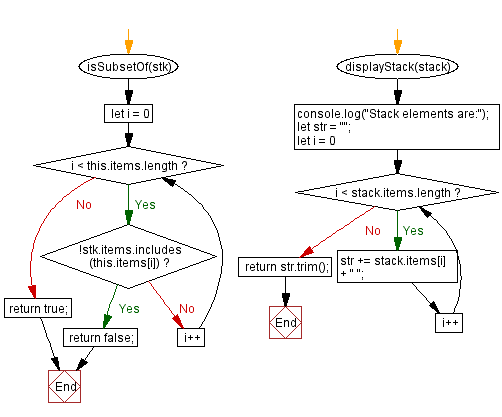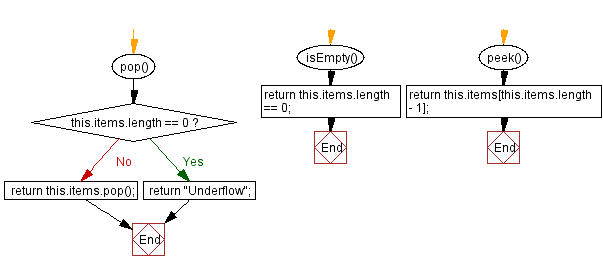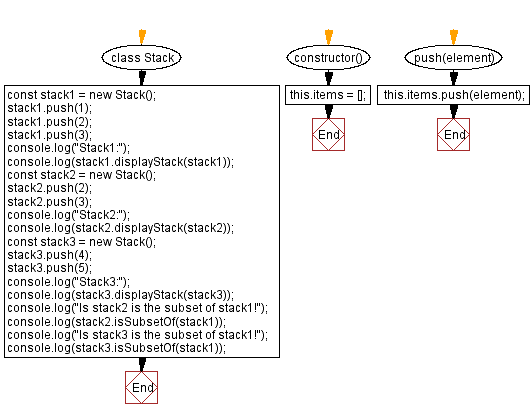# JavaScript Exercises: Check if a stack is a subset of another stack

## JavaScript Stack: Exercise-26 with Solution

Write a JavaScript program that implements a stack and checks if a stack is a subset of another stack.

Sample Solution:

JavaScript Code:

``````class Stack {
constructor() {
this.items = [];
}

push(element) {
this.items.push(element);
}

pop() {
if (this.items.length == 0) {
return "Underflow";
}
return this.items.pop();
}

isEmpty() {
return this.items.length == 0;
}

peek() {
return this.items[this.items.length - 1];
}

isSubsetOf(stk) {
for (let i = 0; i < this.items.length; i++) {
if (!stk.items.includes(this.items[i])) {
return false;
}
}
return true;
}
displayStack(stack) {
console.log("Stack elements are:");
let str = "";
for (let i = 0; i < stack.items.length; i++)
str += stack.items[i] + " ";
return str.trim();
}
}
const stack1 = new Stack();
stack1.push(1);
stack1.push(2);
stack1.push(3);
console.log("Stack1:");
console.log(stack1.displayStack(stack1));
const stack2 = new Stack();
stack2.push(2);
stack2.push(3);
console.log("Stack2:");
console.log(stack2.displayStack(stack2));
const stack3 = new Stack();
stack3.push(4);
stack3.push(5);
console.log("Stack3:");
console.log(stack3.displayStack(stack3));
console.log("Is stack2 is the subset of stack1!");
console.log(stack2.isSubsetOf(stack1));
console.log("Is stack3 is the subset of stack1!");
console.log(stack3.isSubsetOf(stack1));
```
```

Sample Output:

```Stack1:
Stack elements are:
1 2 3
Stack2:
Stack elements are:
2 3
Stack3:
Stack elements are:
4 5
Is stack2 is the subset of stack1!
true
Is stack3 is the subset of stack1!
false
```

Flowchart:Live Demo:

See the Pen javascript-stack-exercise-26 by w3resource (@w3resource) on CodePen.

Improve this sample solution and post your code through Disqus

Stack Previous: Create a copy of the stack.
Stack Exercises Next: Checks if two stacks are equal.

What is the difficulty level of this exercise?

Test your Programming skills with w3resource's quiz.

﻿